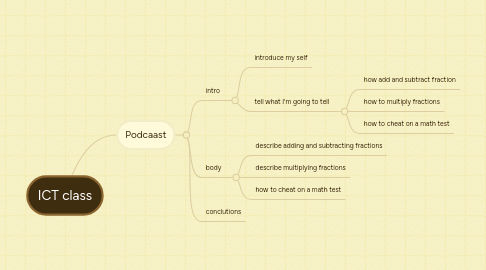# ICT class

RW
Rj Woll

Get Started. It's FreeICT class by## 1. Podcaast

### 1.1. intro

1.1.1. introduce my self

1.1.2. tell what I'm going to tell

1.1.2.1. how add and subtract fraction

1.1.2.2. how to multiply fractions

1.1.2.3. how to cheat on a math test

### 1.2. body

1.2.1. describe adding and subtracting fractions

1.2.2. describe multiplying fractions

1.2.3. how to cheat on a math test